# Overview

The Random Forest Classification Forecast Processor is used to estimate a categorical variable column based on the random forest classifier. A random forest is a meta estimator that fits a number of decision tree classifiers on various sub-samples of the dataset and uses averaging to improve the predictive accuracy and control overfitting.

# Input

The processor requires two input datasets. The first input port (the one on the left) corresponding to the training dataset (this data should be already labeled). The second input port (the one on the right) corresponding to the test dataset. Both datasets must contain a categorical variable column (The variable to predict).

# Output

The Random Forest Classification Forecast Processor returns four different output tables:

• Forecast Output: The original dataset with an additional column that contains the predicted labels. The column name is specified in the third field in the processor's configuration.
• Debug Output: Shows which category was replaced by the fallback category mentioned in the processor's configuration. In case of no replacements the Debug Output is empty. It can also be empty if the corresponding configuration is toggled off by the last configuration field.
• Mapping Output: Creates an RDD that contains all the categories for each feature that were mapped to fake values.
• Feature Importance Output: Returns the variable importance ranking for all independent variables within a two column table. It shows which of the independent variables were most important in predicting the dependent variable.

In addition, other outputs can be viewed within the "result" tab in the processor:

• RANDOM FOREST TREES: The different random trees used, along with each tree's weight.
• RANDOM FOREST INDEPENDENT IMPORTANCES: The bar chart for the different importance fractions assigned to each independent variable.
• RANDOM FOREST DEBUG: The generic JSON Object for the debug result.

# Example

In this example, the input dataset is the iris flower dataset. Each row of the dataset represents an iris flower, including its species and dimensions of its botanical parts, sepal and petal, in centimeters. The goal here is to predict the flower's specie using the available dimensions.

## Example Configuration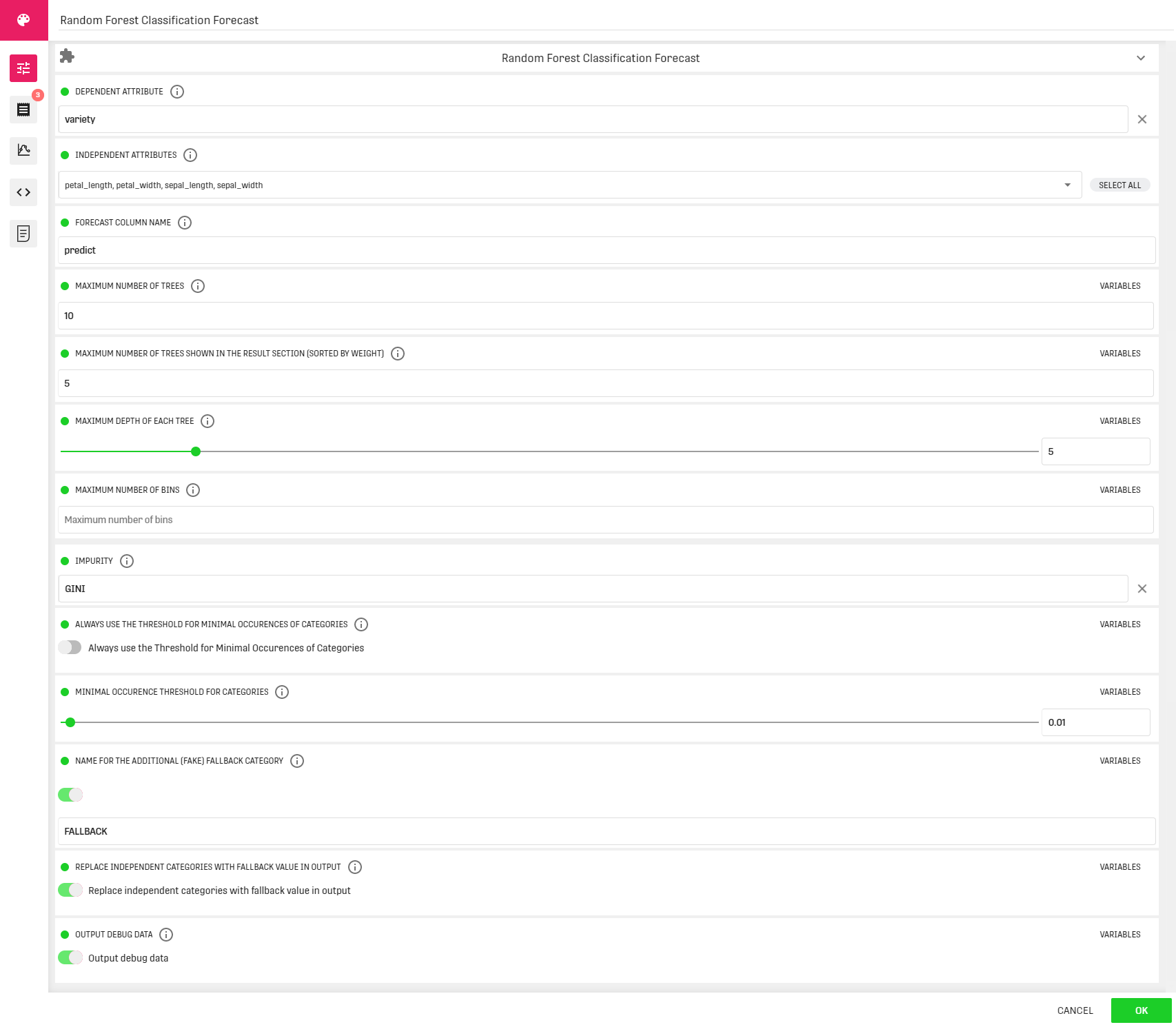Here we use all the remaining variables as independent.

## Workflow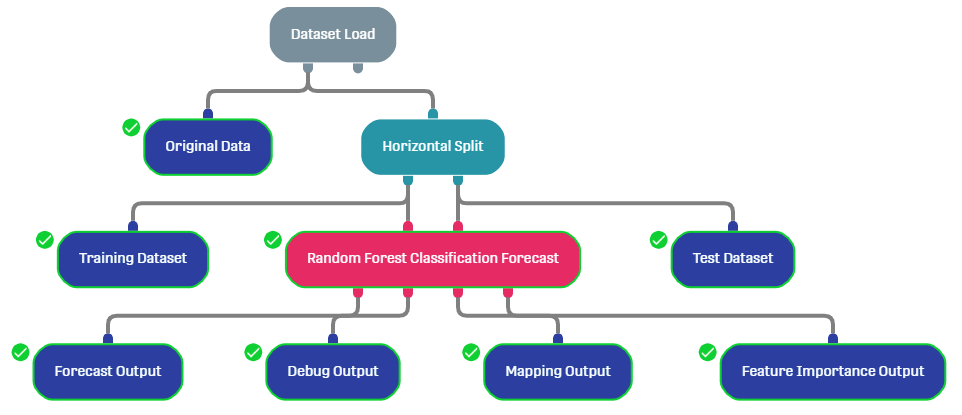The dataset is split into a training dataset (90%) and a test dataset (10%) using the Horizontal split processor.

## Result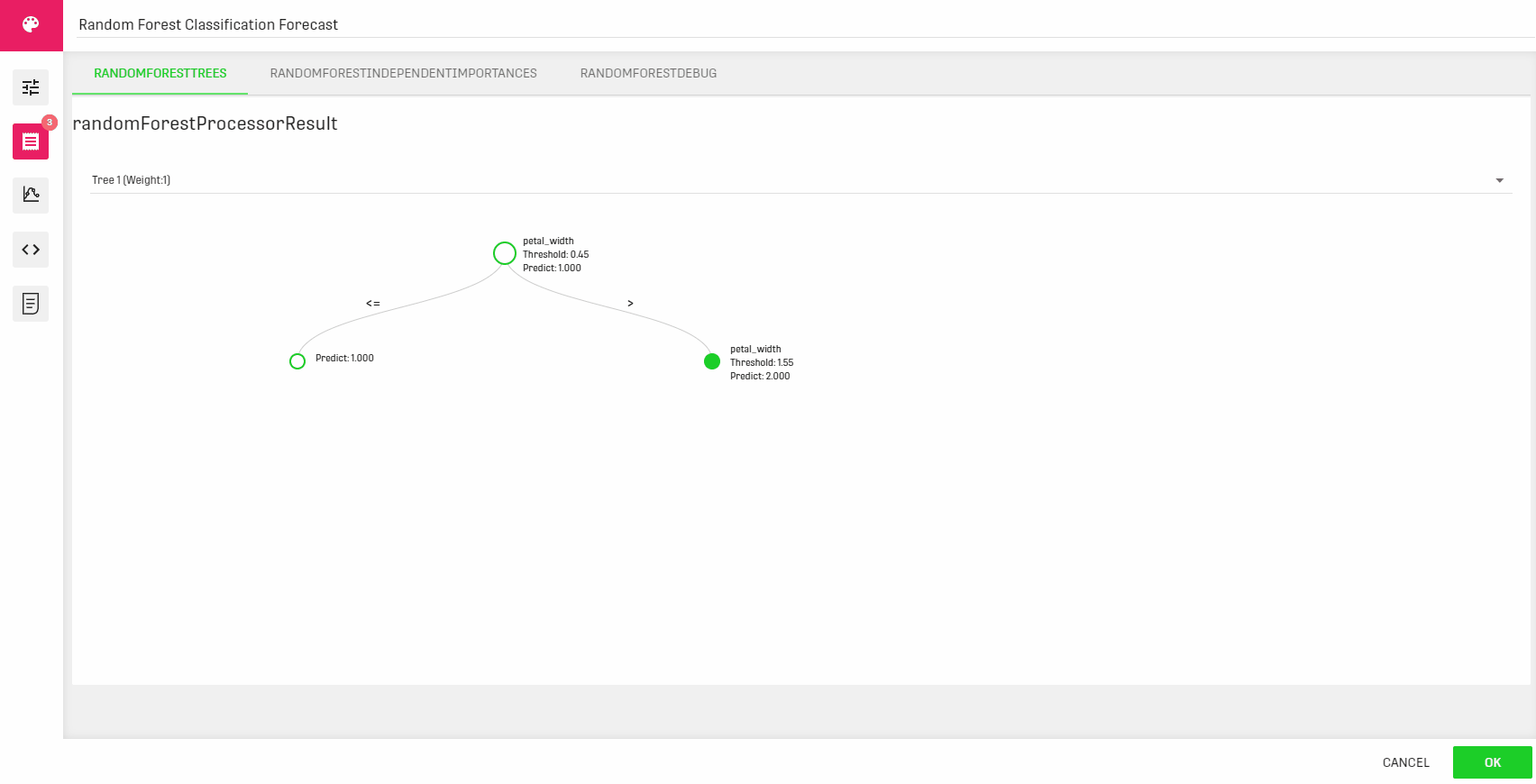Within the processor, the different decision trees used are displayed along with the corresponding weights.
A bar chart of the independent variables presenting the different importance fractions is also displayed in the second tab.

• Forecast Output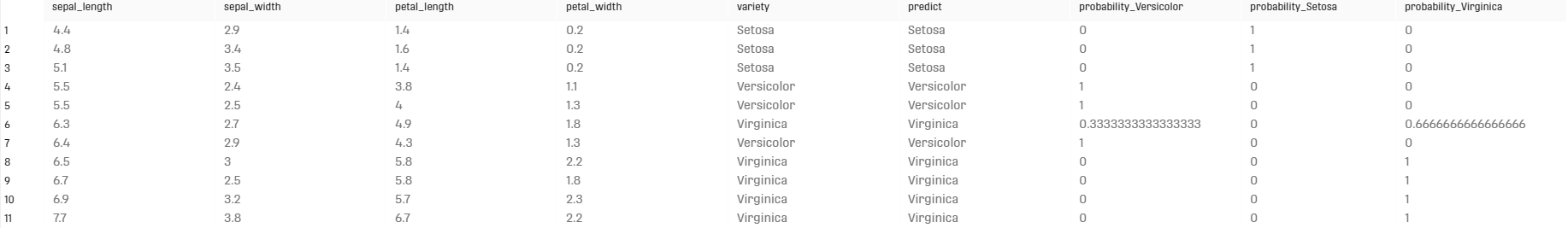Along with the predicted label, the Forecast Output table gives the different probabilities of all the available labels.

• Feature Importance Output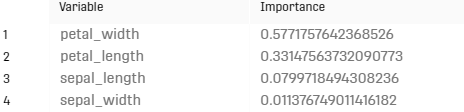Relevant Info: The user can use Forecast metrics Processors such as the Forecast Metrics Processor and the Forecast Metrics For For each Processor to evaluate the performance of the random forest processor

# Related Articles

Decision Tree Classification Forecast Processor

Random Forest Regression Forecast Processor